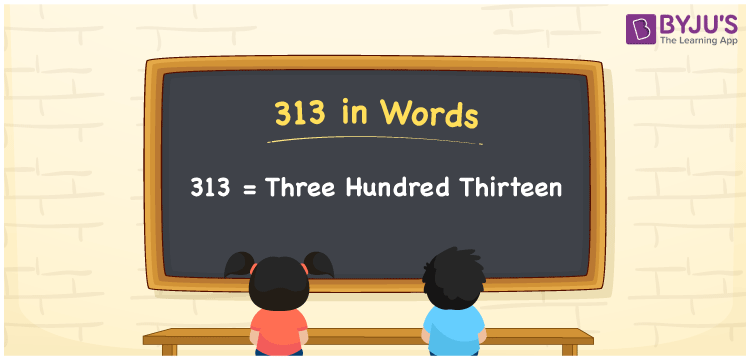# 313 in Words

We can write 313 in words as Three hundred thirteen. It is easy to convert the number 313 into words with the help of a place value chart. As we know, the word form of single and double-digit numbers can be written quickly, but to obtain the word form of three and more digit numbers, we must use the place value chart. In this article, you will learn how to convert 313 into words, along with some interesting facts about 313.

 313 in words Three hundred thirteen Three hundred thirteen in Numbers 313

## 313 in English Words

We generally use the English alphabet to write numbers in words. Thus, we spell 313 in English words as “Three hundred thirteen”.## How to Write 313 in Words?

The below table illustrates the place value chart for the given number, i.e., 313. Let’s learn how to derive the word form of 313.

 Hundreds Tens Ones 3 1 3

Here, ones = 3, tens = 1, hundreds = 3

Let us expand the above digits with respect to their place values.

3 × Hundred + 1 × Ten + 3 × One

= 3 × 100 + 1 × 10 + 3 × 1

= 300 + 10 + 3

= Three hundred + Thirteen {since ten + three = thirteen}

= Three hundred thirteen

Therefore, 313 in words is written as Three hundred thirteen.

We know that 313 is a natural number that precedes 314 and succeeds 312.

313 in words – Three hundred thirteen

Is 313 an even number? – No

Is 313 an odd number? – Yes

Is 313 a prime number? – Yes

Is 313 a composite number? – No

Is 313 a perfect square number? – No

Is 313 a perfect cube number? – No

## Frequently Asked Questions on 313 in Words

Q1

### How do you write 313 in words?

We can write the number 313 in words as Three hundred thirteen.
Q2

### Write the value of 313 + 500 in words.

313 + 500 = 813 Thus, the value of 313 + 500, i.e., 813 in words is Eight hundred thirteen.
Q3

### How to write Rs. 313 in words on a cheque?

On a cheque, we generally write Rs. 313 in words as “Three hundred thirteen rupees only”.